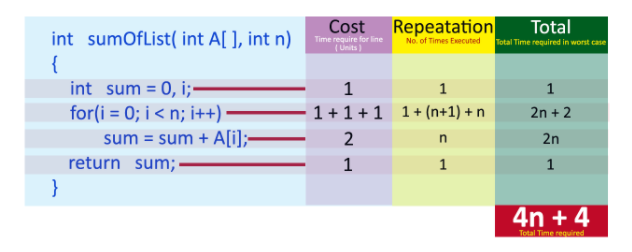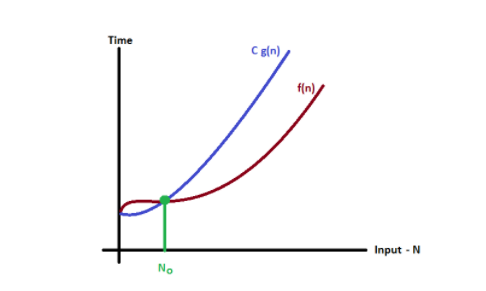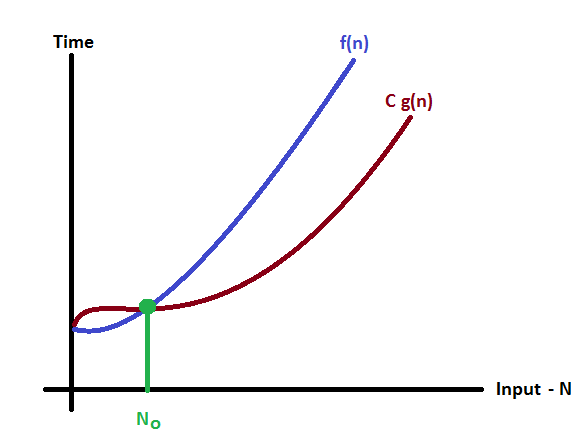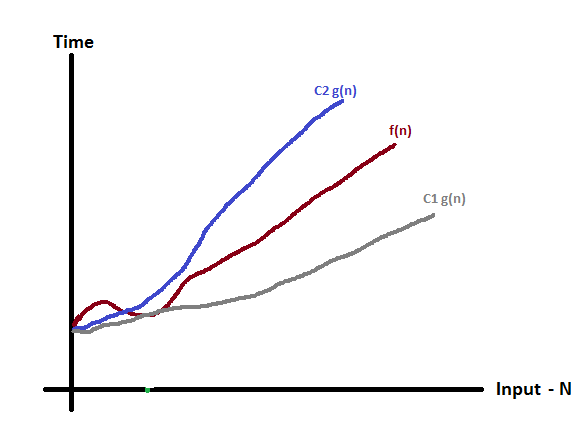# Chapter 05 : Complexity & Types | Asymptotic Analysis | Asymptotic Notation

### Complexity

​​

An essential aspect to data structures is algorithms. Data structures are implemented using algorithms. An algorithm is a procedure that you can write as a C function or program or any other language. An algorithm states explicitly how the data will be manipulated.

### Algorithm Complexity

Algorithmic complexity is concerned about how fast or slow particular algorithm performs. We define complexity as a numerical function T (n) - time versus the input size n.

Usually, the efficiency or running time of an algorithm is stated as a function relating the input length to the number of steps, known as time complexity, or volume of memory, known as space complexity.

We want to define time taken by an algorithm without depending on the implementation details. But you agree that T (n) does depend on the implementation! A given algorithm will take different amounts of time on the same inputs depending on such factors as: processor speed; instruction set, disk speed, brand of compiler and etc. The way around is to estimate efficiency of each algorithm asymptotically. We will measure time T (n) as the number of elementary "steps" (defined in any way), provided each such step takes constant time.

What to Measures?

• CPU Time

• Memory

• Number of steps

• Number of particular operations

• Asymptotic complexity

### Space Complexity

When we design an algorithm to solve a problem, it needs some computer memory to complete its execution. For any algorithm, memory is required for the following purposes...

• Memory required to store program instructions

• Memory required to store constant values

• Memory required to store variable values

• And for few other things

Definition:

Total amount of computer memory required by an algorithm to complete its execution is called as space complexity of that algorithm

Generally, when a program is under execution it uses the computer memory for THREE reasons. They are as follows...

• Instruction Space: It is the amount of memory used to store compiled version of instructions.

• Environmental Stack: It is the amount of memory used to store information of partially executed functions at the time of function call.

• Data SpaceIt is the amount of memory used to store all the variables and constants.

To calculate the space complexity, we must know the memory required to store different datatype values (according to the compiler). For example, the C Programming Language compiler requires the following...

• 2 bytes to store Integer value,

• 4 bytes to store Floating Point value,

• 1 byte to store Character value,

• 6 (OR) 8 bytes to store double value

Example 1

Consider the following piece of code...

int square(int a)

{

return a*a;

}

In above piece of code, it requires 2 bytes of memory to store variable 'a' and another 2 bytes of memory is used for return value

In above piece of code, it requires 2 bytes of memory to store variable 'a' and another 2 bytes of memory is used for return value.

That means, totally it requires
4 bytes of memory to complete its execution. And this 4 bytes of memory is fixed for any input value of 'a'. This space complexity is said to be Constant Space Complexity.

Definition:

If any algorithm requires a fixed amount of space for all input values then that space complexity is said to be Constant Space Complexity

Example 2

int sum(int A[], int n)

{

​​ ​​​​ int sum = 0, i;

​​ ​​​​ for(i = 0; i < n; i++)

​​ ​​ ​​ ​​ ​​​​ sum = sum + A[i];

​​ ​​​​ return sum;

}

In above piece of code it requires

'n*2' bytes of memory to store array variable 'a[]'
2 bytes of memory for integer parameter 'n'
4 bytes of memory for local integer variables 'sum' and 'i' (2 bytes each)
2 bytes of memory for return value.

That means, totally it requires '2n+8' bytes of memory to complete its execution. Here, the amount of memory depends on the input value of 'n'. This space complexity is said to be Linear Space Complexity.

If the amount of space required by an algorithm is increased with the increase of input value, then that space complexity is said to be Linear Space Complexity

### Time Complexity

Every algorithm requires some amount of computer time to execute its instruction to perform the task. This computer time required is called time complexity.

Definition:

The time complexity of an algorithm is the total amount of time required by an algorithm to complete its execution.

Generally, running time of an algorithm depends upon the following...

• Whether it is running on Single processor machine or Multi processor machine.

• Whether it is a 32 bit machine or 64 bit machine

• Read and Write speed of the machine.

• The time it takes to perform Arithmetic operations, logical operations, return value and assignment operations etc.,

• Input data

To calculate time complexity of an algorithm, we need to define a model machine. Let us assume a machine with following configuration.

• Single processor machine

• 32 bit Operating System machine

• It performs sequential execution

• It requires 1 unit of time for Arithmetic and Logical operations

• It requires 1 unit of time for Assignment and Return value

• It requires 1 unit of time for Read and Write operations

Example 1

int sum(int a, int b)

{

​​ ​​​​ return a+b;

}

In above sample code, it requires 1 unit of time to calculate a+b and 1 unit of time to return the value. That means, totally it takes 2 units of time to complete its execution. And it does not change based on the input values of a and b. That means for all input values, it requires same amount of time i.e. 2 units.

If any program requires fixed amount of time for all input values then its time complexity is said to be Constant Time Complexity.

Example 2

int sum(int A[], int n)

{

​​ ​​​​ int sum = 0, i;

​​ ​​​​ for(i = 0; i < n; i++)

​​ ​​ ​​ ​​ ​​​​ sum = sum + A[i];

​​ ​​​​ return sum;

}Cost is the amount of computer time required for a single operation in each line.
Repetition is the amount of computer time required by each operation for all its repetitions.
Total is the amount of computer time required by each operation to execute.

So above code requires
'4n+4' Units of computer time to complete the task. Here the exact time is not fixed. And it changes based on the n value. If we increase the n value then the time required also increases linearly.

Totally it takes
'4n+4' units of time to complete its execution and it is Linear Time Complexity.

Definition:

If the amount of time required by an algorithm is increased with the increase of input value then that time complexity is said to be Linear Time Complexity

### Asymptotic Analysis

Asymptotic notation of an algorithm is a mathematical representation of its complexity

Asymptotic analysis of an algorithm refers to defining the mathematical foundation/framing of its run-time performance. Using asymptotic analysis, we can very well conclude the best case, average case, and worst case scenario of an algorithm.

Usually, the time required by an algorithm falls under three types

• Best Case − Minimum time required for program execution, or the minimum number of steps taken on any instance of size a.

• Average Case − Average time required for program execution or an average number of steps taken on any instance of size a.

• Worst Case − Maximum time required for program execution or the maximum number of steps taken on any instance of size a.

### Asymptotic Notation

Following are the commonly used asymptotic notations to calculate the running time complexity of an algorithm.

• Ο Notation (Big Oh)

• Ω Notation (omega)

• θ Notation (theta)

Ο Notation (Big Oh)

The notation Ο (n) is the formal way to express the upper bound of an algorithm's running time. It measures the worst case time complexity or the longest amount of time an algorithm can possibly take to complete.

A good basic unit of computation for comparing the summation algorithms shown earlier might be to count the number of assignment statements performed to compute the sum. In the function sumOfN, the number of assignment statements is 1(theSum=0) plus the value of n (the number of times we perform (theSum = theSum+i). We can denote this by a function, call it T, where T (n) =1+n. The parameter n is often referred to as the “size of the problem,” and we can read this as “T (n) is the time it takes to solve a problem of size n, namely 1+n steps.”

a=5

b=6

c=10

for i in range(n):

​​ ​​​​ for j in range(n):

​​ ​​ ​​ ​​ ​​​​ x = i * i

​​ ​​ ​​ ​​ ​​​​ y = j * j

​​ ​​ ​​ ​​ ​​​​ z = i * j

for k in range(n):

​​ ​​​​ w = a*k + 45

​​ ​​​​ v = b*b

d = 33

The number of assignment operations is the sum of four terms. The first term is the constant 3, representing the three assignment statements at the start of the fragment. The second term is 3n2, since there are three statements that are performed n2 times due to the nested iteration. The third term is 2n, two statements iterated n times. Finally, the fourth term is the constant 1, representing the final assignment statement.

​​ This gives us   T (n) =3+3n2+2n+1

​​ T (n) =3n2+2n+4

As another example, suppose that for some algorithm, the exact number of steps is T (n) =5n2+27n+1005. When n is small, say 1 or 2, the constant 1005 seems to be the dominant part of the function. However, as n gets larger, the n2 term becomes the most important. In fact, when n is really large, the other two terms become insignificant in the role that they play in determining the final result. ​​ Again, to approximate T (n) as n gets large, we can ignore the other terms and focus on 5n2. In addition, the coefficient 5 becomes insignificant as n gets large. We would say then that the function T (n) has an order of magnitude f (n) =n2, or simply that it is O (n2).

Big - Oh Notation (O)

Consider function f(n) the time complexity of an algorithm and g(n) is the most significant term. If f(n) <= C g(n) for all n >= n0, C > 0 and n0 >= 1. Then we can represent f(n) as O(g(n)).

f(n) = O(g(n))

Consider the following graph drawn for the values of f(n) and C g(n) for input (n) value on X-Axis and time required is on Y-AxisIn above graph after a particular input value n0, always C g(n) is greater than f(n) which indicates the algorithm's upper bound.

Example

Consider the following f(n) and g(n)...
f(n) = 3n + 2
g(n) = n
If we want to represent f(n) as O(g(n)) then it must satisfy f(n) <= C x g(n) for all values of C > 0 and n0>= 1

f(n) <= C g(n)
3n + 2 <= C n

Above condition is always TRUE for all values of C = 4 and n >= 2.
By using Big - Oh notation we can represent the time complexity as follows...
3n + 2 = O(n)

Big - Omege Notation (Ω)

Big - Omega notation is used to define the lower bound of an algorithm in terms of Time Complexity.

That means Big - Omega notation always indicates the minimum time required by an algorithm for all input values. That means Big - Omega notation describes the best case of an algorithm time complexity.

Consider function f(n) the time complexity of an algorithm and g(n) is the most significant term. If f(n) >= C x g(n) for all n >= n0, C > 0 and n0 >= 1. Then we can represent f(n) as Ω(g(n)).

f(n) = Ω(g(n))

Consider the following graph drawn for the values of f(n) and C g(n) for input (n) value on X-Axis and time required is on Y-AxisIn above graph after a particular input value n0, always C x g(n) is less than f(n) which indicates the algorithm's lower bound.

Example

Consider the following f(n) and g(n)...
f(n) = 3n + 2
g(n) = n
If we want to represent f(n) as Ω(g(n)) then it must satisfy f(n) >= C g(n) for all values of C > 0 and n0>= 1

f(n) >= C g(n)
3n + 2 <= C n

Above condition is always TRUE for all values of C = 1 and n >= 1.
By using Big - Omega notation we can represent the time complexity as follows...
3n + 2 = Ω(n)

Big - Theta Notation (Θ)

Big - Theta notation is used to define the average bound of an algorithm in terms of Time Complexity.

That means Big - Theta notation always indicates the average time required by an algorithm for all input values. That means Big - Theta notation describes the average case of an algorithm time complexity.

Consider function f(n) the time complexity of an algorithm and g(n) is the most significant term. If C1g(n) <= f(n) >= C2 g(n) for all n >= n0, C1, C2 > 0 and n0 >= 1. Then we can represent f(n) as Θ(g(n)).

f(n) = Θ(g(n))

Consider the following graph drawn for the values of f(n) and C g(n) for input (n) value on X-Axis and time required is on Y-AxisIn above graph after a particular input value n0, always C1 g(n) is less than f(n) and C2 g(n) is greater than f(n)which indicates the algorithm's average bound.

Example

Consider the following f(n) and g(n)...
f(n) = 3n + 2
g(n) = n
If we want to represent f(n) as Θ(g(n)) then it must satisfy C1 g(n) <= f(n) >= C2 g(n) for all values of C1, C2 > 0 and n0>= 1

C1 g(n) <= f(n) >= C2 g(n)
C1 n <= 3n + 2 >= C2 n

Above condition is always TRUE for all values of C1 = 1, C2 = 4 and n >= 1.
By using Big - Theta notation we can represent the time complexity as follows.

3n + 2 = Θ(n)